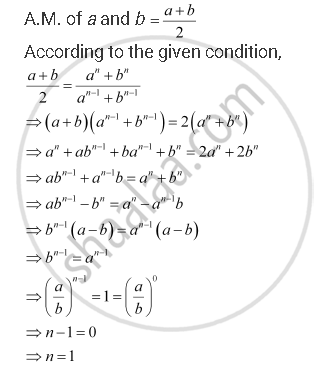Share

# If (A^N + B^N)/(A^(N-1) + B^(N-1)) is the Arithmetic Mean Between a and B, Then Find the Value of N. - Mathematics

#### Question

if (a^n + b^n)/(a^(n-1) + b^(n-1)) is the A.M. between a and b, then find the value of n.

#### SolutionIs there an error in this question or solution?

#### APPEARS IN

NCERT Solution for Mathematics Textbook for Class 11 (2018 (Latest))
Chapter 9: Sequences and Series
Q: 15 | Page no. 185

#### Video TutorialsVIEW ALL 

Solution If (A^N + B^N)/(A^(N-1) + B^(N-1)) is the Arithmetic Mean Between a and B, Then Find the Value of N. Concept: Arithmetic Progression (A.P.).
S